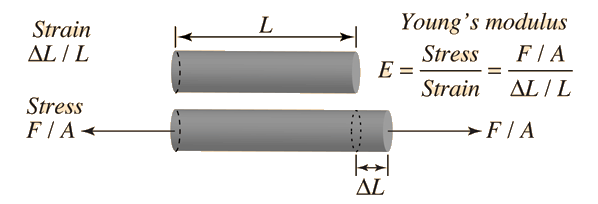# PhysicsFind force with young modulus

#### Cbarker1

##### Active member
Q: Calculate the force (in N) a piano tuner applies to stretch a steel piano wire 8.50 mm, if the wire is originally 0.850 mm in diameter and 1.35 m long. (Young's modulus for steel is 20.0 1010 Pa.)

Work F=Y*A*change in L/L_0

#### skeeter

##### Well-known member
MHB Math Helper
$L = 1.35 \, m$

$\Delta L = 8.5 \times 10^{-3} \, m$

$r = 4.25 \times 10^{-4} \, m$

$E=2.0 \times 10^{11} \, N/m^2$

$F = \dfrac{E \cdot \pi r^2 \cdot \Delta L}{L}$